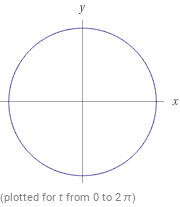Search IntMath
Close

# Circle Theorems in Geometry: An IntroductionCircle theorems in geometry refer to the various properties and relationships between circles and angles formed by chords, tangents, and secants of a circle. Not only are circle theorems important in geometry, but they also appear in trigonometry, algebra, and even calculus. In this article, we will explore the basics of circle theorems and how to use them in proofs.

## Understanding the Basics of Circle Theorems

A circle theorem is a statement about the properties of a circle. These theorems involve angles and lengths of lines that can be used to calculate central angles and arcs. Examples of commonly used circle theorems include the Chord-Chord Theorem, Chord-Tangent Theorem, and the Secant-Secant Theorem.

The Chord-Chord Theorem states that when two chords cross in a circle, the product of the lengths of one pair of opposite chords is equal to the product of the lengths of the other pair of opposite chords. For example, if chord AB is 8 and chord CD is 6, then the product of chord AB and chord CD is equal to the product of chord AD and chord CB, which is 48.

The Chord-Tangent Theorem states that the product of the length of the chord and the length of the tangent of a circle is equal to the square of the radius. In other words, if chord AB is 8 and the length of the tangent at point A is 10, then the product of chord AB and the length of the tangent is equal to the square of the radius, which is 80.

The Secant-Secant Theorem states that the product of the lengths of two secant lines is equal to the product of the lengths of the two segments that are cut by the secant lines. For example, if secant line AC is 10 and secant line BD is 12, then the product of the two secant lines is equal to the product of the lengths of the two segments (AB and CD) that are cut by the secant lines, which is 120.

## How to Use Circle Theorems in Proofs

In order to use circle theorems in proofs, you need to be able to identify the type of theorem that applies to the problem you are trying to solve. Once you have identified the type of theorem, you can then use the theorem to prove that the statement is true.

For example, if you are trying to prove that the product of the lengths of two chords is equal to the product of the lengths of the two segments that are cut by the chords, then you can use the Chord-Chord Theorem to prove your statement is true.

It is important to remember that in order for a circle theorem to be true, all of the angles and lengths must be given. This means that if you are given only the lengths of two chords, but not the lengths of the two segments that are cut by the chords, then the Chord-Chord Theorem will not apply.

## Practice Problems

1. If the radius of a circle is 5 and the length of the chord is 8, what is the length of the tangent?

2. If the length of two secant lines are 10 and 12, what is the product of the lengths of the two segments that are cut by the secant lines?

3. If chord AB is 3 and chord CD is 5, what is the product of chord AB and chord CD?

4. If the radius of a circle is 8 and the length of the tangent is 12, what is the length of the chord?

5. If chord AB is 6 and chord CD is 8, what is the product of chord AD and chord CB?

6. If secant line AC is 8 and secant line BD is 10, what is the product of the two secant lines?

## Summary

In this article, we explored circle theorems in geometry, which are statements about the properties of circles. We discussed the basics of circle theorems, including the Chord-Chord Theorem, Chord-Tangent Theorem, and the Secant-Secant Theorem. We also discussed how to use circle theorems in proofs. Finally, we provided some practice problems so you can test your knowledge.

Circle theorems are important in geometry, trigonometry, algebra, and calculus. Understanding circle theorems and how to use them in proofs can help you solve a variety of problems. We hope this article has been helpful in introducing you to the basics of circle theorems.

## FAQ

### What is a Circle Theorem?

A Circle Theorem is a statement about circles that is proved using geometry. It usually involves relationships between the diameter, radius, chords, tangents, secants, arcs, and other elements of the circles.

### What is the Inscribed Angle Theorem?

The Inscribed Angle Theorem states that when two chords intersect in a circle, the measure of the inscribed angle formed is equal to one-half the measure of its intercepted arc.

## Problem SolverThis tool combines the power of mathematical computation engine that excels at solving mathematical formulas with the power of GPT large language models to parse and generate natural language. This creates math problem solver thats more accurate than ChatGPT, more flexible than a calculator, and faster answers than a human tutor. Learn More.